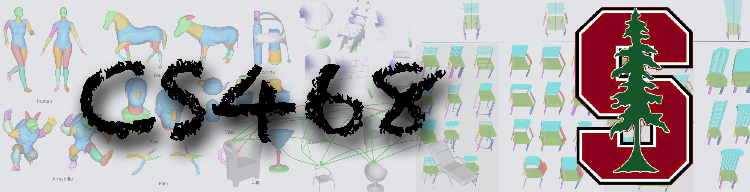# Assignment 1: Shape Retrieval

You can use the following base code that already implements a lot of basic datastructures.

1. Sample points on a mesh
1.1  Pick every vertex as a sample point. Let's call it a point set {V}.
1.2  Generate 100,000 random points, where probability of a point on a face
is proportional to the face area. Let's call it a point set {P_rnd}
1.3  Iterative Farthest Points: start with a random point, select a point
in {P_rnd} that is as far as possible from the existing set of points. Construct 1000 points

2 Shape Descriptors
2.1  Compute D2 Shape Descriptor for each mesh (pick your favorite set of points in 1.)
2.2  Compute D2 Shape Descriptor with geodesic distances (hint: use Dijkstra algorithm)
2.3  Align all pairs of shapes with an ICP algorithm. Pick Identity as the initial alignment.
hint: you might find R3Kdtree.cpp and RNSvd.cpp to be useful for this project.
Also, take a look at this writeup.

3 Evaluation and Applications
3.1  For every descriptor run a retrieval experiment (create an html page that would show
top 5 most similar models for each query shape and corresponding L2 distance between their shape
descriptors, use average point-to-point distance for descriptor 2.3).
3.2  Draw precision / recall curves as you vary the number of retrieved models.
Assume there are 3 classes of shapes: planes, birds, and humans.
3.3  Create a confusion matrix: record how often one class was confused with another).
Suppose the first retrieved shape defines the classification of the query shape.

4 Report
Summarize your findings in a single report.pdf (please, have a coherent structure and
write in full sentences). Include intermediate results in your report (e.g. point samples,
example histograms for some shapes, etc). Also describe evaluation results, point out interesting
success cases and limitations, and discuss potential improvements.

Along with the report include the retrieval results as html pages (one page per descriptor),